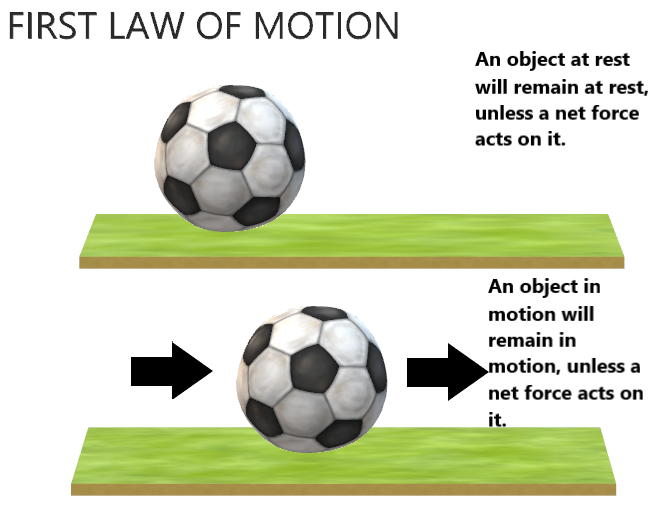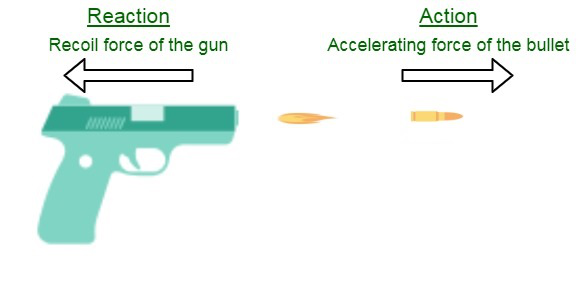# CBSE Class 9 Science Chapter 9 Revision Notes

Chapter 9: Force and Laws of Motion Revision Notes

## BALANCED AND UNBALANCED FORCES

Balanced Forces

• When two or more forces are applied to the same object at the same time, the net force is created. The net force is the sum of all applied forces.
• The force exert in one direction is added to the force you exert in the opposing direction. Because the forces are balanced and equal.

Unbalanced Forces

• A force is delivered in one direction, followed by either a smaller or bigger force in the opposite direction, or no force at all in the opposite direction.
• Forces that are unbalanced Will a chair move if I press on one side of it with a force of 50 N and you push on the opposite side with a force of 25 N? Which direction is it going to go? The most force is delivered in this direction. What is the magnitude of the net force? 50 degrees north, 25 degrees north.

## LAWS OF MOTION

Newton’s First LawSource:

Unless acted upon by an unbalanced force, an object at rest will remain at rest and an object in motion will remain in motion at constant velocity.

Unless acted upon by an unbalanced force, an object at rest will remain at rest, and an object in motion will remain in motion at constant velocity.

Newton’s Second Law

• “The object will accelerate if the net force on it is not zero. The direction of acceleration coincides with the direction of net force. The amplitude of the acceleration is proportional to the applied net force and inversely proportional to the object’s mass.”

• For all of this, mathematical symbols give a useful shorthand:

F= ma

The Effect of Mass

• A force applied to a car will have a different effect than a force applied to a pencil. Because it has more mass, an automobile resists acceleration far more than a pencil does.
• An object’s acceleration is determined not only by how strongly you push it, but also by how hard it resists being pushed.
• What influence does mass have on acceleration? This, too, proves to be pretty straightforward (I wonder why…). An object with twice the mass will accelerate at half the rate for the same force. The same force would cause one-third the acceleration if it had three times the mass. One-fourth of the acceleration is equal to four times the mass, and so on.
• An inverse proportion or inverse variation is a form of relationship between quantities (double one, receive half the other). To put it another way:
• The Second Law of Motion by Newton An object’s acceleration is determined by both force and mass. As a result of the contact force that occurs during the collision, if the colliding objects have uneven mass, they will have asymmetrical accelerations.

Newton’s Third LawSource:

• Newton’s Third Law states that there is an equal and opposite reaction to every action.
• The phrase “action…reaction” refers to the fact that forces usually occur in pairs. (Conversations are interactions between people, while forces are interactions between objects.)
• It is impossible to have single, isolated forces. The “action force” and the “reaction force” are the two forces at work.
• For a variety of reasons, these names are unfortunate:
• In a given interaction, either the “action” or “reaction” force can be used.
• At the same moment, the action and response forces exist.

What Does “Equal” Mean?

• Both forces are same in size. They are of the same magnitude. Both forces are present at the same moment. Both begin and end at the same time. In terms of time, they are on par.
• The term “opposite” refers to the fact that the two forces always act in opposing directions – completely opposite each other.
• The third law of motion by Newton There are two forces acting on the two interacting objects in every interaction. The force acting on the first object is equal to the force acting on the second object. The force on the first object is directed in the opposite direction as the force on the second object. Equal and opposing action-reaction force pairings are always present.
• Collisions between two objects were subjected to Newton’s third law of motion. When two objects collide, they both experience forces that are equal in magnitude but opposite in direction. As a result of these forces, one item accelerates (gains momentum) while the other slows down (lose momentum). The forces acting on the two objects are equal in magnitude, according to Newton’s third law.

## MASS AND INERTIA

• The tendency of an object to resist any change in its motion is known as inertia. Unless acted upon by an imbalanced force, an object will continue to move at the same speed and in the same direction.
• A bowling ball with inertia and mass rolling down the road would ultimately come to a halt. Friction is a force that causes the ball to stop or slow down due to an imbalanced force. The ball would continue to roll if there was no friction.
• The amount of matter in a thing is measured by its mass. A tennis ball has less mass than a bowling ball. The bigger an object’s mass, the greater its inertia.
• The measurement of inertia is mass.

## CONSERVATION OF MOMENTUM

The vector sum of the momenta before and after an impact must be equal in a closed system.

Source:

]]>